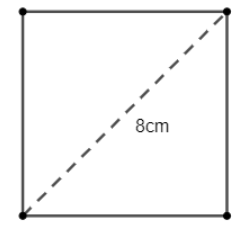QuestionAnswers

# If the diagonal of a square is 8 cm, then its area is(a) $4c{{m}^{2}}$ (b) $16c{{m}^{2}}$(c) $24c{{m}^{2}}$(d) $32c{{m}^{2}}$Hint: Use the property that area of the square is equal to half of the square of the length of the diagonal of the square, i.e., area of the square is $\dfrac{{{l}^{2}}}{2}$ , where l is the length of the diagonal. So, just put the length of the diagonal in the formula and solve to get the answer.

Let us start the solution to the above question by drawing a representative diagram of the situation given in the figure.Now we know that the area of the square is equal to half of the square of the length of the diagonal of the square.
$\therefore \text{ Area of the square = }\dfrac{{{\left( diagonal \right)}^{2}}}{2}$
Now, it is given that the length of the diagonal of the square is 8cm. So, if we put this in our equation, we get
$\text{Area of the square = }\dfrac{{{8}^{2}}}{2}$
Now, we know that the square of 8 is equal to 64.
$\text{Area of the square = }\dfrac{64}{2}=32c{{m}^{2}}$
Therefore, the area of the square whose diagonal is 8 cm in length is equal to 32 sq cm.

Hence, the answer to the above question is option (d).

Note: We could have also solved the above question using the property that the diagonal of a square is $\sqrt{2}$ times the length of its side followed by the use of the formula that the area of the square is equal to the square of the length of its side.

Square Root of 8CBSE Class 8 Maths Chapter 6 - Squares and Square Roots FormulasArea of Square FormulaWhere There is a Will There is a Way EssayArea of a Sector of a Circle FormulaThe Difference Between an Animal that is A Regulator and One that is A ConformerArea of a Rhombus FormulaHow to Find Square Root of a NumberSquare Root of 576Square Root of 144Important Questions for CBSE Class 6 English A Pact with The Sun Chapter 8 - A Pact with the SunImportant Questions for CBSE Class 8 Maths Chapter 8 - Comparing QuantitiesImportant Questions for CBSE Class 6 English Honeysuckle Chapter 8 - A Game of ChanceImportant Questions for CBSE Class 8 Maths Chapter 6 - Squares and Square RootsCBSE Class 8 Science Reaching The Age of Adolescence WorksheetsImportant Questions for CBSE Class 8 English Honeydew Chapter 8 - A Short Monsoon DiaryImportant Questions for CBSE Class 8 Science Chapter-8 Cell - Structure and FunctionsImportant Questions for CBSE Class 8 Social Science Our Past 3 Chapter 4 - Tribals, Dikus and the Vision of a Golden AgeImportant Questions for CBSE Class 8 English Honeydew Chapter 6 - This is Jody’s FawnImportant Questions for CBSE Class 8 MathsCBSE Class 10 Hindi A Question Paper 2020Hindi A Class 10 CBSE Question Paper 2009Hindi A Class 10 CBSE Question Paper 2015Hindi A Class 10 CBSE Question Paper 2016Hindi A Class 10 CBSE Question Paper 2012Hindi A Class 10 CBSE Question Paper 2010Hindi A Class 10 CBSE Question Paper 2007Hindi A Class 10 CBSE Question Paper 2013Hindi A Class 10 CBSE Question Paper 2008Hindi A Class 10 CBSE Question Paper 2014NCERT Solutions for Class 8 Maths Chapter 6 Squares and Square RootsRS Aggarwal Class 8 Mathematics Solutions for Chapter-18 Area of a Trapezium and a PolygonNCERT Solutions for Class 9 English Moments Chapter 8 - A House is Not a HomeRS Aggarwal Solutions Class 8 Chapter-18 Area of a Trapezium and a Polygon (Ex 18B) Exercise 18.2RS Aggarwal Solutions Class 8 Chapter-18 Area of a Trapezium and a Polygon (Ex 18C) Exercise 18.3RS Aggarwal Solutions Class 8 Chapter-18 Area of a Trapezium and a Polygon (Ex 18A) Exercise 18.1RS Aggarwal Solutions Class 8 Chapter-8 Linear Equations (Ex 8B) Exercise 8.2RS Aggarwal Solutions Class 8 Chapter-8 Linear Equations (Ex 8A) Exercise 8.1RD Sharma Class 8 Solutions Chapter 8 - Division of Algebraic Expressions (Ex 8.6) Exercise 8.6RD Sharma Class 8 Solutions Chapter 8 - Division of Algebraic Expressions (Ex 8.4) Exercise 8.4